Home » Comprehensive Introduction to merging in SAS

# Comprehensive Introduction to merging in SAS

In my previous article, “Combining data sets in SAS – Simplified“, we discussed three methods to combine data sets – appending, concatenating and Interleaving. In this article, we will look at the most common and frequently used method of combining data sets – MERGING or JOINING.

### The need for joining / merging datasets:

Before jumping into the details, let us understand why we actually need joining / merging. Whenever we have some information split and available into two or more data sets and we want to combine them into a single dataset, we need to merge / join these tables. One of the main things to be kept in mind is that the merging should be based on common criteria or field. For example, In a retail company, we have a daily Transaction table (Table contains Products Detail, Sales Detail and Customers Detail) and Inventory table (which has Product Detail and Available quantity). Now, to have the information on Inventory or the availability of a product, what we need to do? Combine the Transaction table with Inventory table based on Product_Code and subtract the sold quantity from available quantity.

Merging / Joining can be of various types and it depends on the business requirement and relationship between data sets. First, let us look at various kinds of relation between data sets can have.

1. When for each value of common variable (say Variable ‘x’) in first data set, second data set has only one matching value for that common variable ‘x’, then it is called ONE-to-ONE relationship.
2. When for value(s) of common variable (say variable ‘y’) in first dataset, other data sets has more than one matching value for that common variable ‘y’, then it is called ONE-to-MANY relationship.
3. When both data sets has multiple entries for same value of common variable then it is called MANY-to-MANY relationship.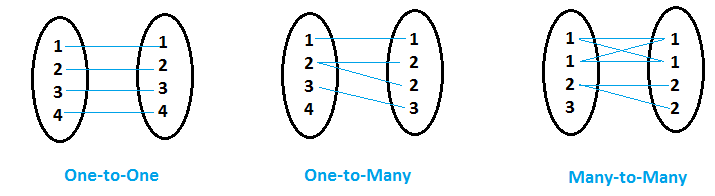In SAS, we can  perform Joining/ Merging through various ways, here we will discuss the most common ways – Data Step and PROC SQL. In Data step, we use Merge statement to perform joins, where as in PROC SQL, we write SQL query. Let us discuss Data step first:

### DATA STEPS

```Syntax:-
Data Dataset;
Merge Dataset1 Dataset2 Dataset3 ...Datasetn;
By CommonVariable1 CommonVariable2......CommonVariablen;
Run;

```

Note:- Data sets must be sorted by common variable(s) and the name, type and length of the common variable should be same for all input data sets.

Let’s look at some scenarios for each of the relationships between input data sets.

### ONE-to-ONE relationship

Scenario:-1 In below input data sets, you can see that there is one to one relationship between these two tables on Student_ID. Now we want to create data set MARKS, where we have all unique student_ids with respective marks of maths and physics. If student_id is not available in Mathematics table, then math_marks should have missing value and vice versa.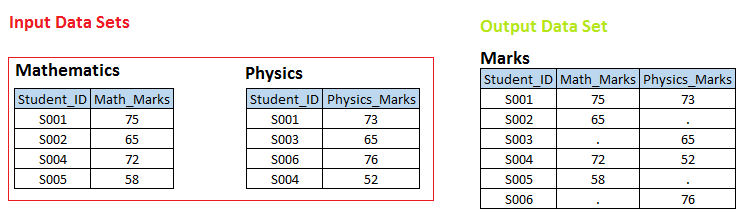Solution using Data Steps:-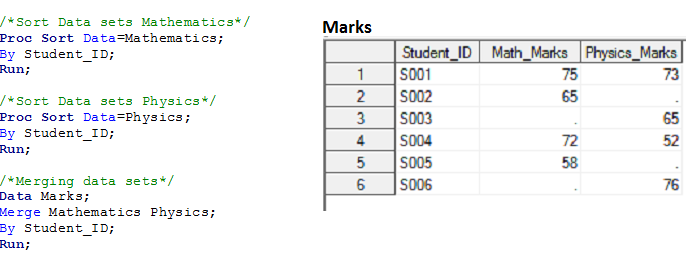How it works:-

1. SAS compares both data sets & creates a PDV (Program data Vector) for all unique variables and initializes them with missing values (Program data Vector is an intermediate between input and output data sets). In current example, it would create a PDV like this: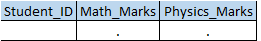2. Reads first observation from input data sets and compares the values of BY variable in both dataset:
1. if values are equal, then it gets compared with the value of BY variable in PDV.
1. if it is not equal, then PDV variables gets reinitialized with missing values and value of current observation is copied to the PDV while the other observsation remains missing
2. If it is equal then PDV variables don’t get reinitialized. Available value of current observation gets updated in the PDV
3. After that, the record pointer moves to the next observation in both data sets and while executing RUN statement, PDV values get transferred to the output data set.
2. If By variable value does not match then observation of data set having lowest value is copied to PDV. The record pointer of data set having lower BY variable value moves to next observation and step 2(a) is repeated again.
3. Above steps repeats till EOF of both data sets is reached.

You can perform a dry run to evaluate the result data set.

Scenario 2:- Based on input data sets of scenario-1, we want to create below output data sets.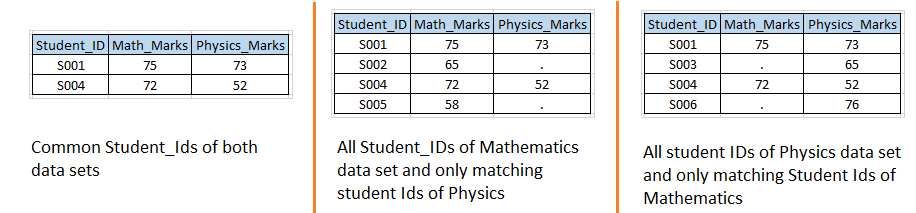Solution using Data Steps:- Let’s write code similar to scenario-1 with IN option.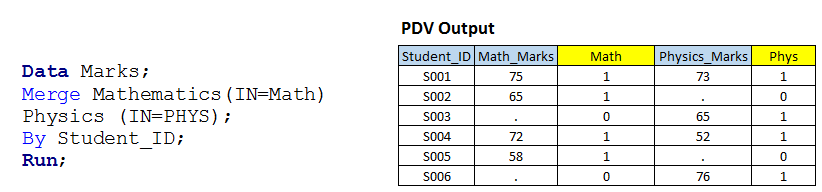Above, you can see that we have used IN option with both input data sets and assigned values of these to temporary variables MATH and PHYS because these are temporary variable so we can not see those in output data set.

I have shown you the table (PDV data) having variable value for all observation along with temporary variables. Now, based on these variable value, we can write a code for sub setting and JOIN operations as we need:

1. If MATH and PHYS both has value 1 then it will create first output data set and called as INNER JOIN.
2. If MATH has 1 then it will create second output data set and called as LEFT JOIN.
3. If PHYS has 1 then it will create third output data set and called as RIGHT JOIN
4. If either MATH and PHYS has 1 then it will work like FULL JOIN, have solved in scenario-1 also.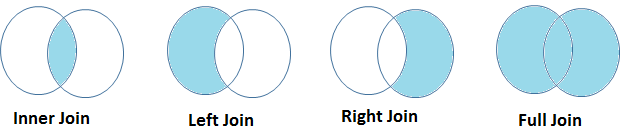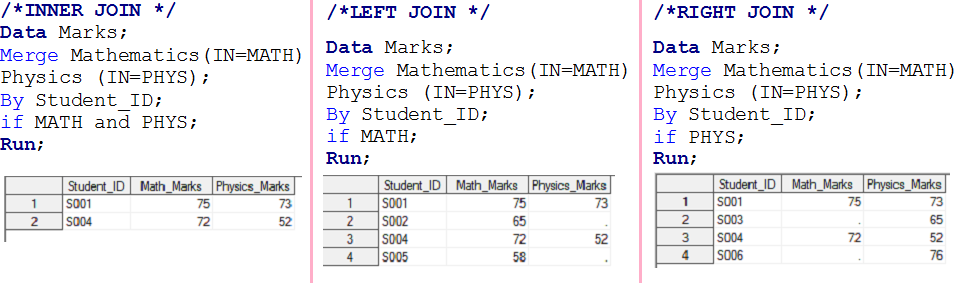ONE-to-MANY relationship

Scenario – 3 Here we have two data sets, Student and Exam and we want to create a output data set Marks.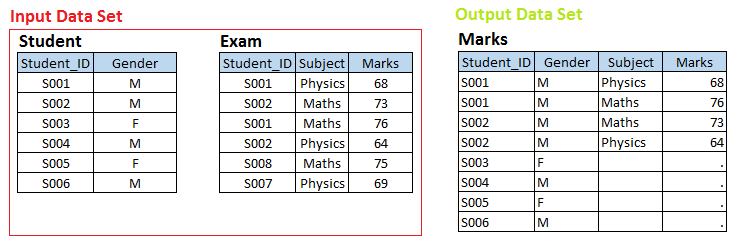Above you look at input data sets, there is one-to-many relationship between Student and Exam. Now if you want to create output data set Marks having individual observation for each exam of students, those belongs to the STUDENT data set i.e Left Join.

Solution using Data Steps:-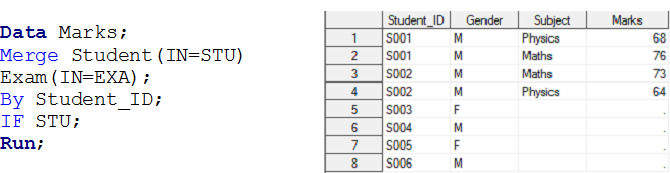In similar way, we can perform operation for Inner, Right and Full join for one-to-many relationship using IN operator.

MANY-to-MANY relationship

Scenario 4: Create a output data sets having all combination based on common field. You can also see that both input data sets has Many-to-Many relationship.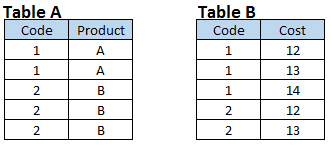Data steps does not perform MANY-to-MANY relationship, because it does not provide output as Cartesian product. When we merge table A and table B using data steps than output is similar as below snapshot.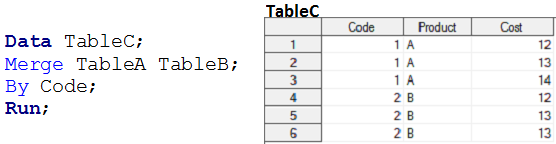Above we have seen, how can we use data steps to merge two or more data sets having any of the relationship except MANY to MANY. Now we will look at PROC SQL methods to have solution for similar requirements.

### PROC SQL

To understand join methodology in SQL, we need to understand Cartesian product first. Cartesian product is a query that has multiple tables in from clause and produces all possible combination of rows from input tables. If we have two tables with 2 and 4 records respectively, then using Cartesian product, we have a table with 2 X 4=8 records.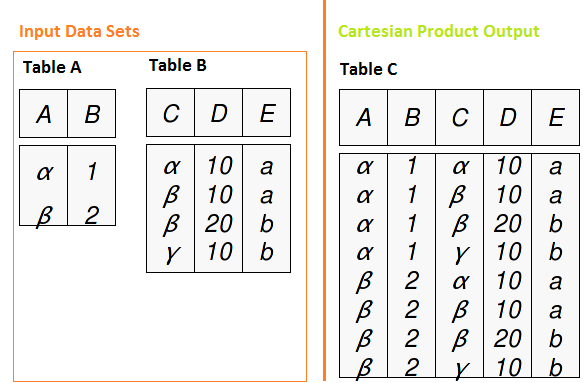SQL joins works for each of the relationship between data sets (One-to-One, One-to-Many and Many-to-Many). Let’s look at how it works with types of joins.

Syntax:-

Select Column-1, Column-2,…Column-n from table1 INNER/ LEFT/ RIGHT/ FULL JOIN table2 ON Join-condition <Other clauses>;

Note:-

1. Tables may or may not be sorted on common variable(s).
2. Common variables name may not be similar but must have similar length and type.
3. Works with a maximum of two tables.

Let’s solve above requirements using PROC SQL.

Scenario -1 :- This was an example of FULL Join, where all Student_IDs was required in output data set with respective MATH and PHYSICS marks.Above in output data set, you can see that Student_ID is missing for those student have appeared only for Physics exam. To solve it we will use a function COALESCE. It returns the value of first non missing argument from given variables.

Syntax:-

COALESCE (argument-1, argument-2,…..argument-n)

Scenario 2 :- This was an example of INNER, Left and right Join. Here we are solving for Inner Join.Similarly we can do for left and right join.

Scenario -3 This was a problem of left join for ONE-to-MANY relationship.Scenario -4 This was a problem of Many-to-MANY relationship. We have already discussed that SQL can produces Cartesian product that contains all combination of records between two tables.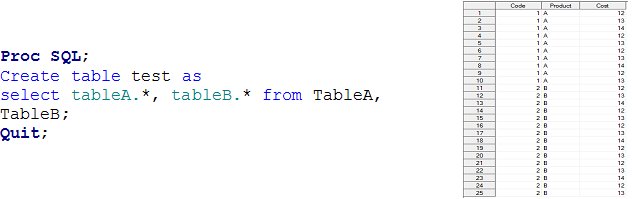Above we have looked at Proc SQL to join/ merge data sets.

### End Note:-

In this series of articles regarding combining data sets in SAS, we looked at various methods to combine data sets like appending, concatenating, interleaving , Merging. Particularly in this article, we discussed that depending on relationship between data sets, various kinds of joins and how can we solve it based on different scenarios. We have used two methods (Data Steps and PROC SQL) to achieve results. We will look at efficiency of these methods in one of the future article.

Have you found this series useful? We have simplified a complex topic like Combining data sets and have tried to present it in understandable manner. If you need any more help with Combining data sets, please feel free to ask your questions through comments below.

P.S. Have you joined Analytics Vidhya Discuss yet? If not, you are missing out on awesome data science discussions. Here are some of the discussions happening on SAS:

### If you like what you just read & want to continue your analytics learning, subscribe to our emails, follow us on twitter or like our facebook page.

You can also read this article on our Mobile APP•Vivek says:

Hi Sunil,

Very good notes on SAS Merging concepts.

Keep up the good work.

Regards,

Vivek

•anukukunuri says:

Hi Sunil,
•avinash says: